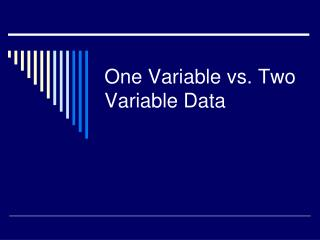DownloadDownload PresentationOne Variable vs. Two Variable Data

# One Variable vs. Two Variable Data

Télécharger la présentation## One Variable vs. Two Variable Data

- - - - - - - - - - - - - - - - - - - - - - - - - - - E N D - - - - - - - - - - - - - - - - - - - - - - - - - - -
##### Presentation Transcript

1. One Variable vs. Two Variable Data

2. One Variable Data One-variable data sets give measures of ONE ATTRIBUTE.

3. Some Examples…….

4. You can recognize one-variable situations when you see: • Tally charts • Frequency tables • Bar graphs • Histograms • Pictographs • Circle graphs

5. Two Variable Data Two-variable data sets give measures of two attributes for each item in a sample.

6. Some Examples…….

7. You can recognize two-variable situations when you see….. • Ordered pairs • Scatter plots • Two-column tables of values

8. One OR Two Variable Data? • Noah researches annual hours of sunshine in Canadian cities • A study compared the length of time children spend playing video games and the time they spend reading

9. Fill in the blanks…… As population of a city increases, increases. As population of a city increases, decreases. As temperature increases, increases.